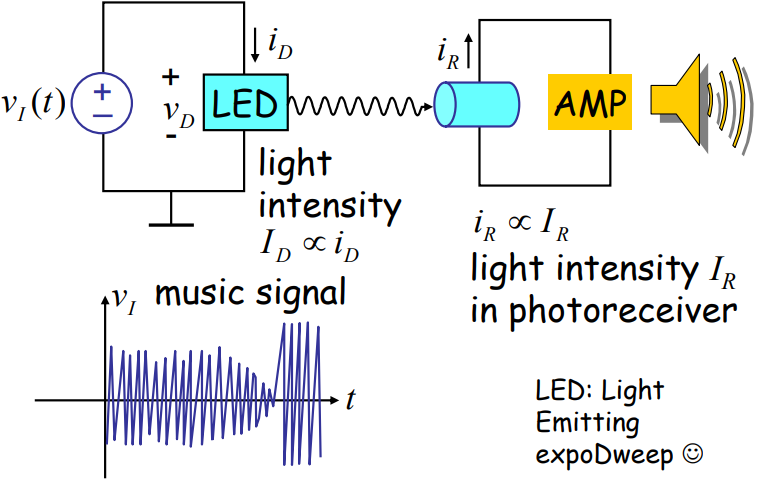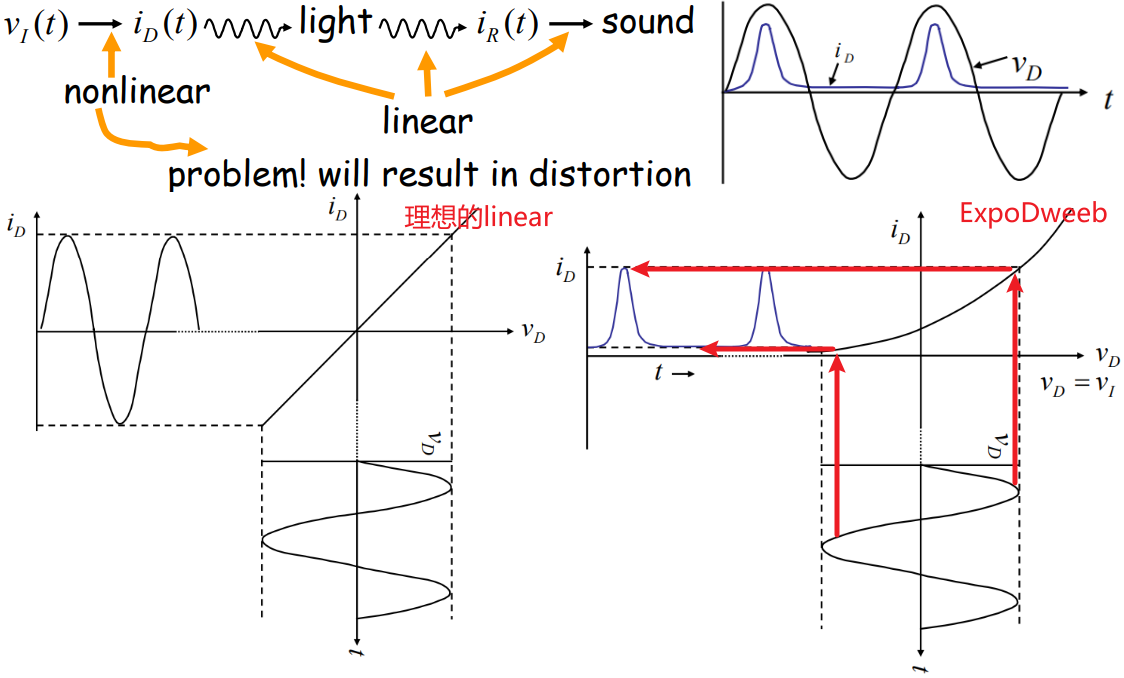## MIT-电路和电子学

• 什么是engineering：ther purposeful use of science
• 思想方法：对于复杂事物，进行层层抽象，然后将问题简化后就能进行后续工作了，即构建复杂系统；
• 工程师不是卖弄数学的人，而是搭建有用的系统的人，并且找到最简单、便利、便宜的方法。工程上的美学就是如何让事情变简单。当问题在数学方面很棘手的时候，我们就应该意识到我们是工程师，让我们找到更简单的方法并运用直觉。
• 本课程的内容：
• Gainful employment of Maxwell's equations
• From electrons to digital gates and op-amps
• 本课程的学习目标：
• Analysis—给出具体电路，说出电路特性；
• Synthesis—给出具体功能，设计电路；
• 英语单词：takeaway  要点 breeze 轻而易举的事 rinky-dink pull rug out shady

## Introduction

### Lumped Abstraction

• lump：块，肿块，一小方块。比如 lump of clay / a lump on my shoulder / a lump of sugar
• 集总元件(Lumped element)：
• 集总元件是指元件大小远小于电路工作频率相对之电磁波波长时，对所有元件之统称，对于信号而言，不论任何时刻，元件特性始终保持固定，与频率无关；
• 信号波长对于元件大小来说是相当长的，所以当信号通过元件时，信号在元件内部每点之变化相当小，可视为相同，所以元件的特性是一体成，因此集总元件之电流与电压关系可以明确定义；下图是无线电波(radio wave)的波长/频率范围，从手机开始，波长可能就比元件短了(微波范围：300MHz-300GHz，1 mm ~ 1 m)，元件就不能看作是集总元件了。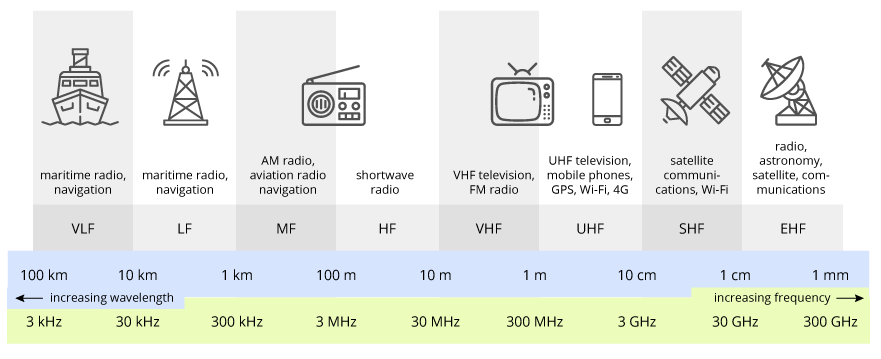• 集总元件，组成的电路模型称为Lumped circuit(集总电路)模型；
• 集总元件，也是单一特征的元件，比如：
• 电阻元件集中表征了某一实际部件或某一段实际电路的能量消耗
• 电感元件集中反映了磁场的物理现象；
• 电容元件集中反映了电场的物理现象；
• outside elements(法拉第定律)   $$\displaystyle\oint E \cdot d l=-\frac{\partial \phi_{B}}{\partial t}=0$$
• inside elements(连续性要求) $$\displaystyle\oint J \cdot d S=-\frac{\partial q}{\partial t}=0$$
• 集总元件构建复杂系统：一个系统的基本组成部分的特性越确定，则复用这些基本组成部分、实现一个系统的难度(成本)就越低。(来自博文确定性) 从集总元件出发，就可以通过层层抽象，构建复杂系统。反面例子就是More Is Different (Philip W. Anderson)。反面例子更具体一点，比如材料科学涉及多尺度的科学问题，不同尺度的问题之间没有简单的联系，即不能用抽象某低尺度的模型来得到更高尺度的材料特性。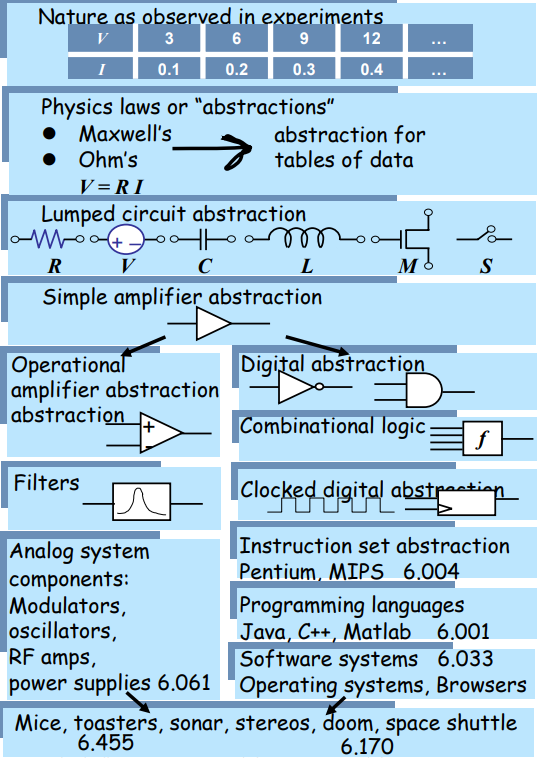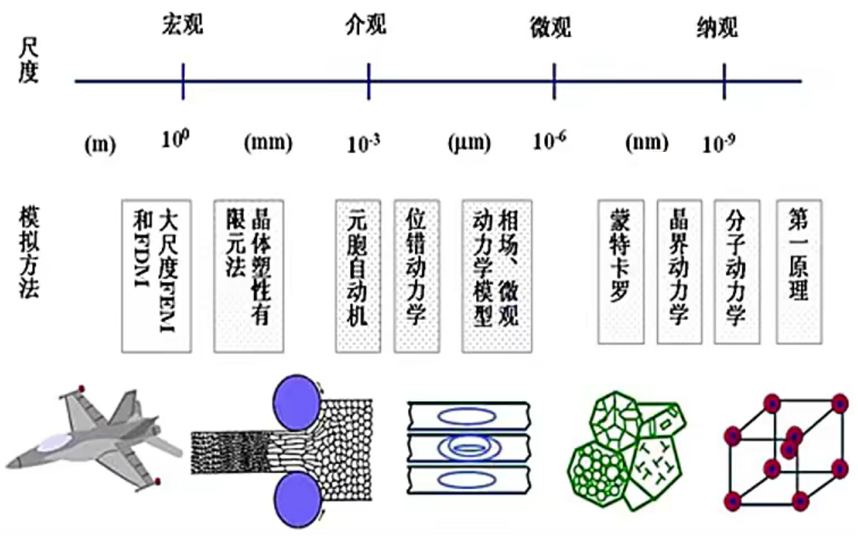• 分布元件(Distributed element)：
• 若元件大小与电路工作频率相对之波长差不多或更大的时候，则当信号通过元件之时，元件本身各点之特性将因信号之变化而有所不同，则此时不能将元件整体视为一特性固定之单一体，而应称为分布元件；
• 分布元件的例子：在微波电路中，传统导线很可能会成为具有电感及电容串并联特性之复杂组合。

Lumped-element model是更广义的概念，比如物理中的质点模型(Point-mass discretization)。更准确的定义如下：The lumped-element model  simplifies the description of the behaviour of spatially distributed physical systems, such as electrical circuits, into a topology consisting of discrete entities that approximate the behaviour of the distributed system under certain assumptions. It is useful in electrical systems (including electronics), mechanical multibody systems, heat transfer, acoustics, etc. This may be contrasted to:
Distributed parameter system in which the behaviour is distributed spatially and cannot be considered as localized into discrete entities. Mathematically speaking, the simplification reduces the state space of the system to a finite dimension, and the partial differential equations (PDEs) of the continuous (infinite-dimensional) time and space model of the physical system into ordinary differential equations (ODEs) with a finite number of parameters.

(1) 集总电路—知乎
(2) Lumped-element model—Wiki

### Basic circuit analysis method

Method 1 — Basic KVL, KCL method of Circuit analysis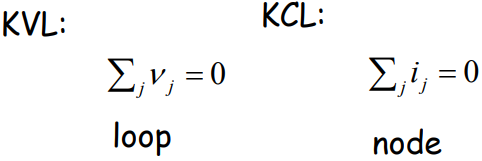Method 2 — Apply element combination rules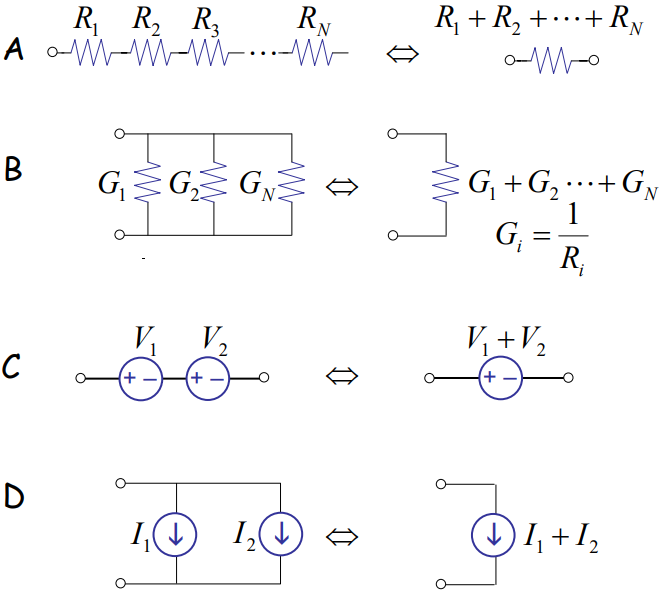Method 3 — Node analysis (Particular application of KVL, KCL method)
(1) Select reference node (ground) from which voltages are measured.
(2) Label voltages of remaining nodes with respect to ground. These are the primary unknowns.
(3) Write KCL for all but the ground node, substituting device laws and KVL.
(4) Solve for node voltages.
(5) Back solve for branch voltages and currents (i.e., the secondary unknowns)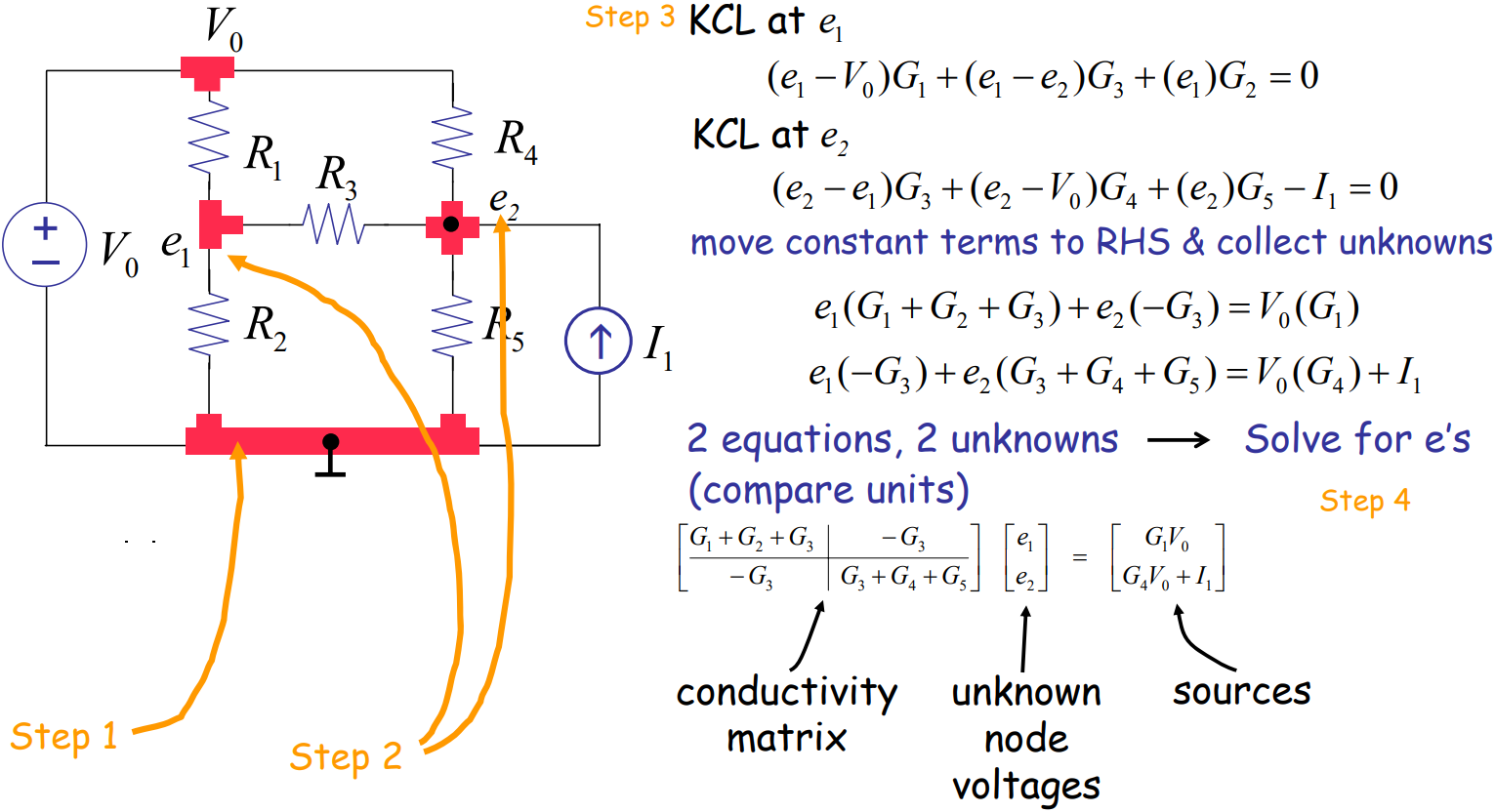### Superposition

Method 4 — Superposition method: The output of a circuit is determined by summing the responses to each source acting alone.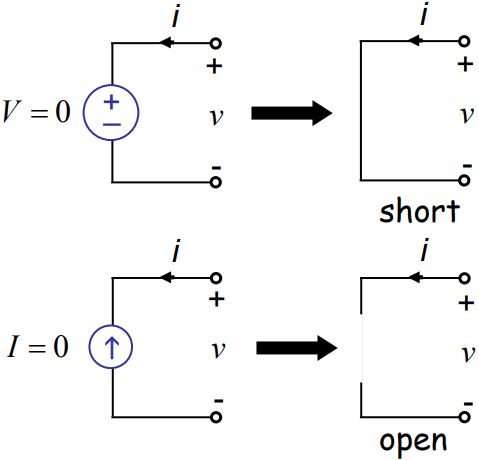其实就是线性叠加原理，在研究某一结点的电压，电路中存在一个恒流电源，一个恒压电源。我们在观察恒流电源对结点电压的影响的时候，无视恒压电源(将其短路)；在观察恒压电源对结点电压的影响的时候，无视恒流电源(将其开路，记成开溜(流)，或者IO)。当然了，我们的研究对象也可以是某一段导线的电流，处理方法还是一致的。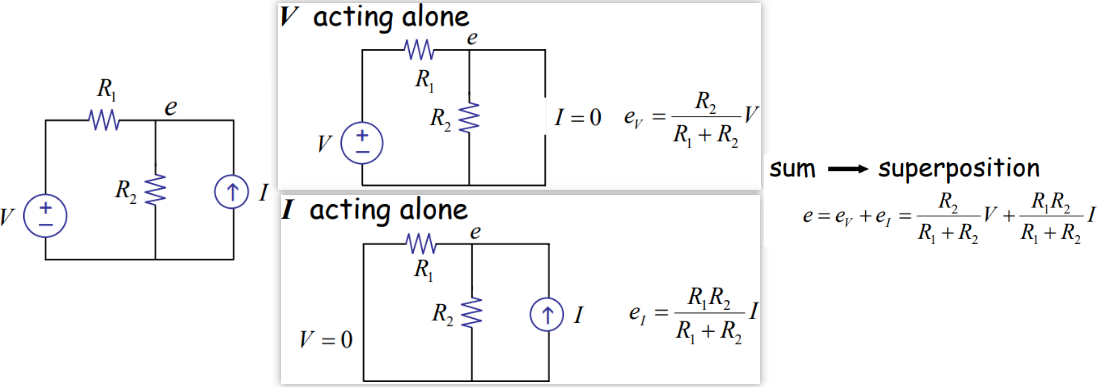### Thévenin and Norton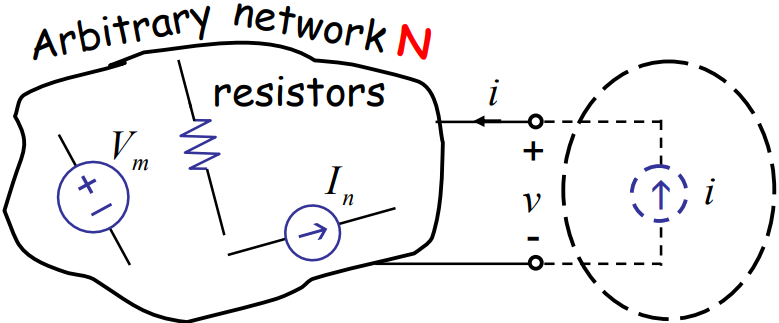• $$m$$个左侧系统内电压源；
• $$n$$个左侧系统内的电流源；
• 右侧外接(系统外)电流源；

• $$\alpha_m$$、$$\beta_n$$和$$R$$其实就是响应系数，响应系数是系统的内在特征，只和电路中的电阻及接线方式有关，和电流源、电压源的大小无关；
• 为了求解每一项的系数，我们让该项以外其他源为零，就可以利用单一元件的V-I特性即可迅速求解出系数，比如为了求解$$R$$，只需要$$\forall_n I_n=0 \quad \forall_m V_m=0$$，然后测量结点的电压，就可以知道左侧图的等效电阻；
• $$\alpha_m$$没有单位(dimensionless quantity)，$$\beta_n$$和$$R$$的单位都是resistance units；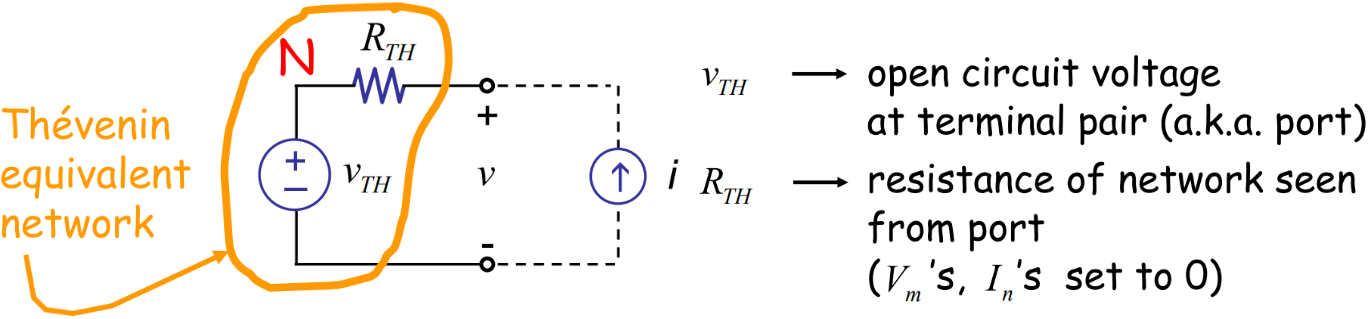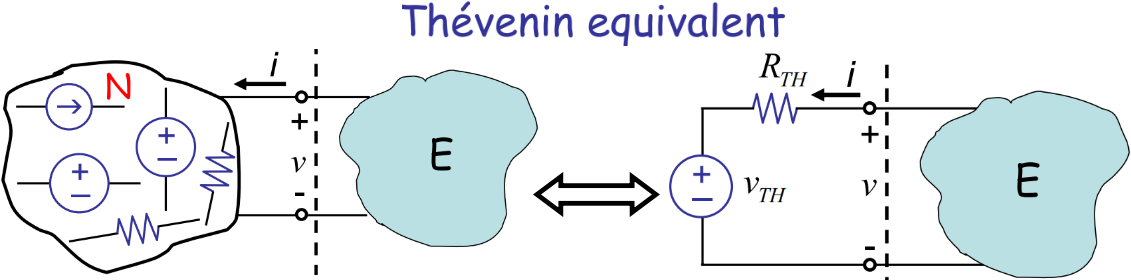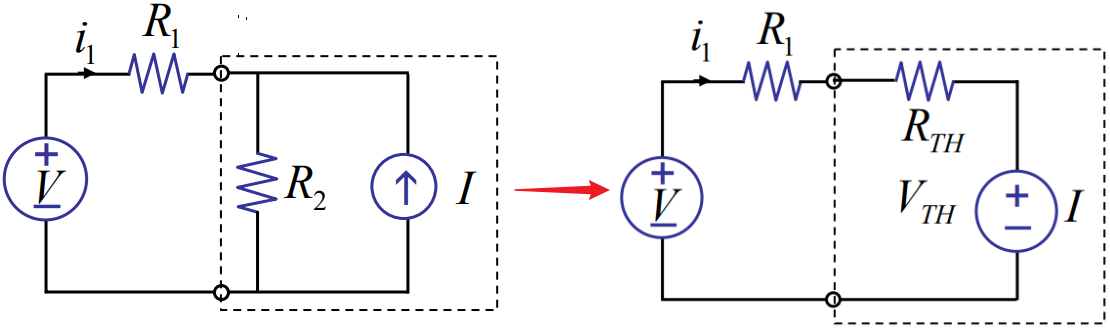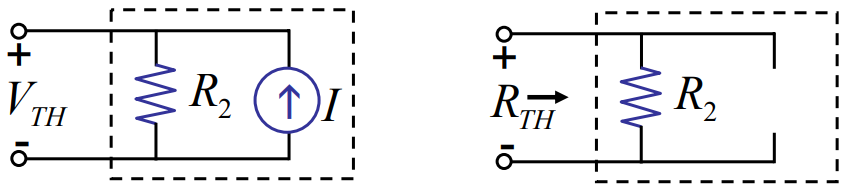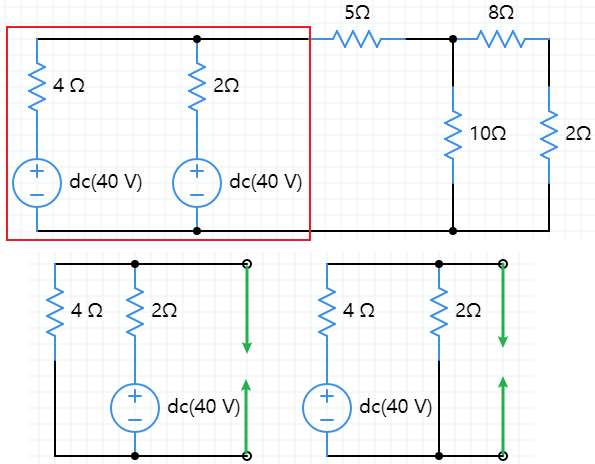(1) 负载电阻为零，计算输出电流，即为$$I_N$$
(2) 负载电阻或者阻抗无穷大，计算输出电压，输出电压除以(1)中的输出电流得到等效电阻$$R_{TH}$$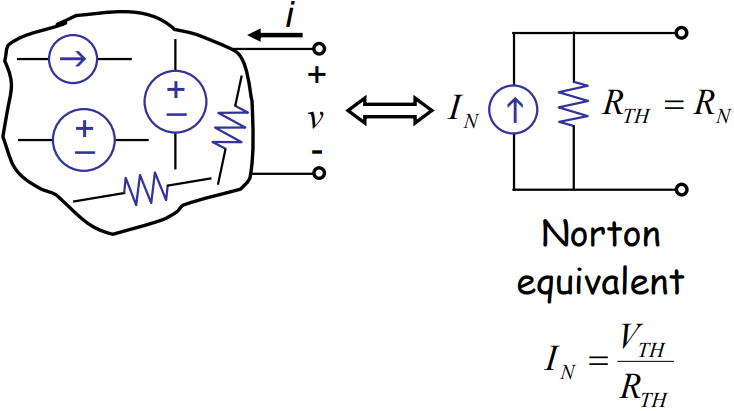## The Digital Abstraction

• 数字信号采用discrete value(也即$$0$$和$$1$$)进行传输，即"低电平"和“高电平”两种状态；
• 判断是高电平还是低电平，是看电压是否落在对应某一个较大的区间。如果最理想的高电平是$$5 \mathrm{~V}$$，低电平是$$0 \mathrm{~V}$$，那么只要在$$2.5 \sim 5 \mathrm{~V}$$范围我们都可以认为是高电平，$$0 \sim 2.5 \mathrm{~V}$$范围我们都可以认为是低电平，即noise margin都是$$2.5 \mathrm{~V}$$；
• numbers larger than $$1$$ can be represented using multiple binary digits and coding, much like using multiple decimal digits (多重十进制数字) to represent numbers greater than $$9$$, the binary number $$101$$ has decimal value $$5$$.
• better noise immunity; lots of noise margin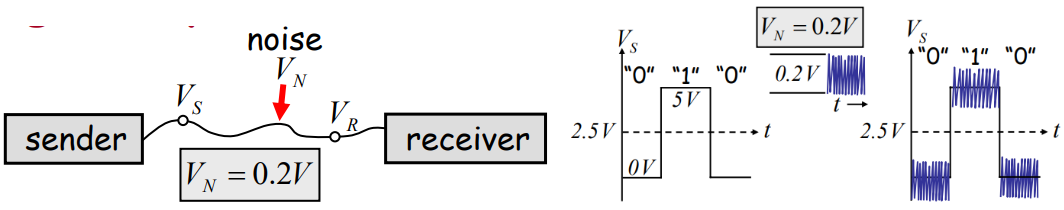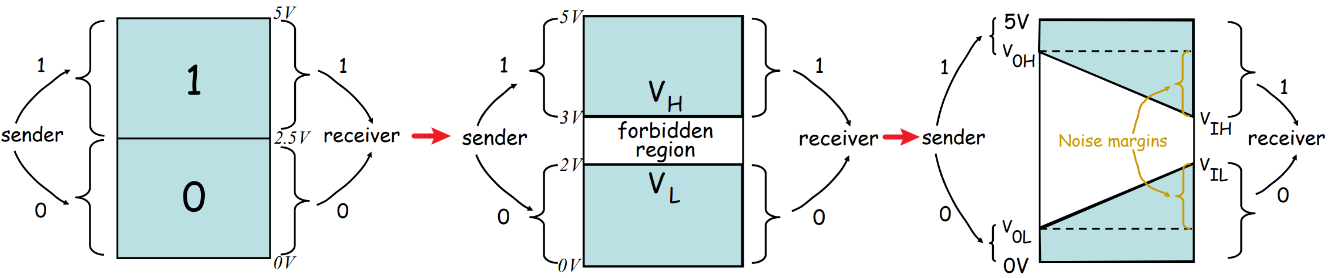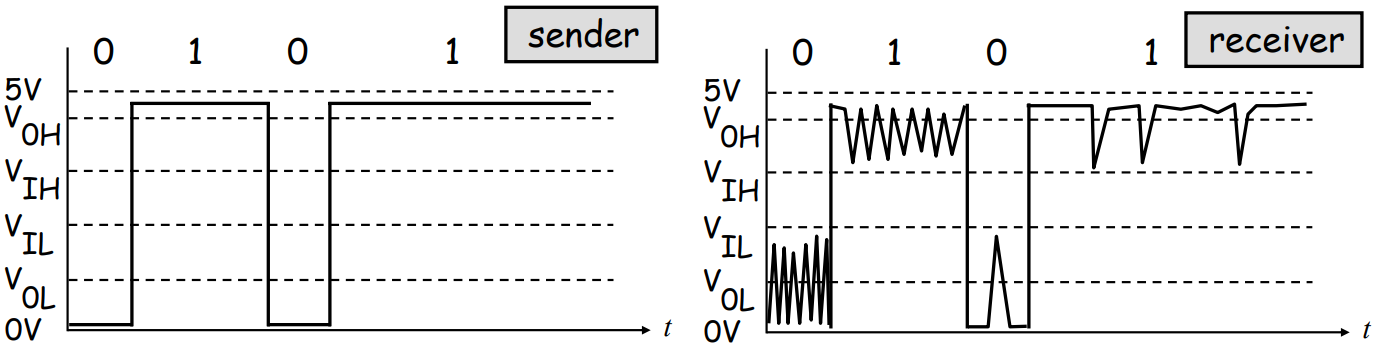• 实际应用中，VOH都比VIH大不少，而且严格要求sender的范围，这样即使有很大的噪音，但是由于noise margin很大，所以仍然能够保证接收端提取出正确的信息。
• Digital systems followstatic discipline(静态规则): if inputs to the digital system meet valid input thresholds, then the system guarantees its outputs will meet valid output thresholds；
• static discipline实际就规定了VOHVOLVIHVIL这四个阈值，所有数字系统都必须遵守，使得彼此之间能够相互通信，比如intel和摩托罗拉想兼容彼此的器件，那么就都要遵守这个静态规则；
• 对消费电子来说，noise margin相对比较小，因为消费者能容忍更多的故障，但是对于医疗领域，或者太空设备，就需要遵守严格得多的标准，这些设备可使用的sender的带宽就很窄，也就有了更大的noise margin。
• 串口通信的TTL高低电平可能会被其他外界电场干扰，进一步提升数字信号传输的稳定性，工业控制中经常使用RS232/RS485总线，参考labview笔记

## Inside the Digital Gate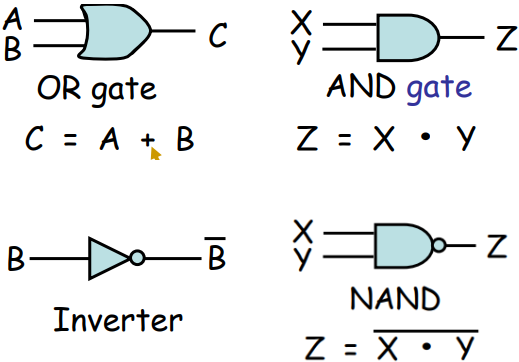Digital Circuits的例子(多个简单gate的组合)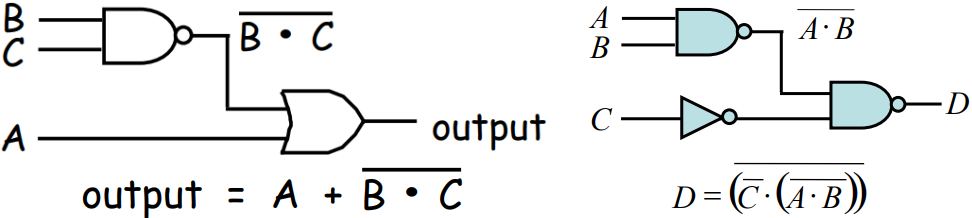Switch的Electrical Analogy：对于传统开关，控制信号来自机械压力。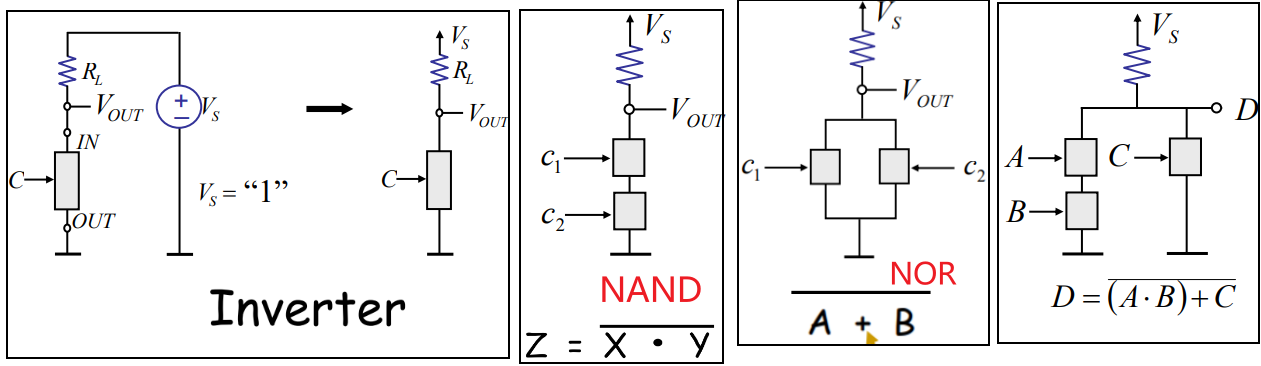MOSFET(Metal-Oxide Semiconductor Field-Effect Transistor): Understand its operation by viewing it as a two-port element. 也叫MOS管。(下图是NMOS的结构)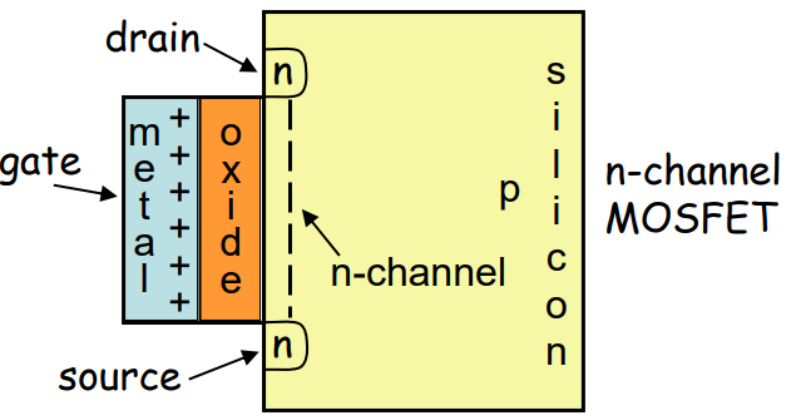Switch modelS model of the MOSFET，忽略了DS之间的电阻。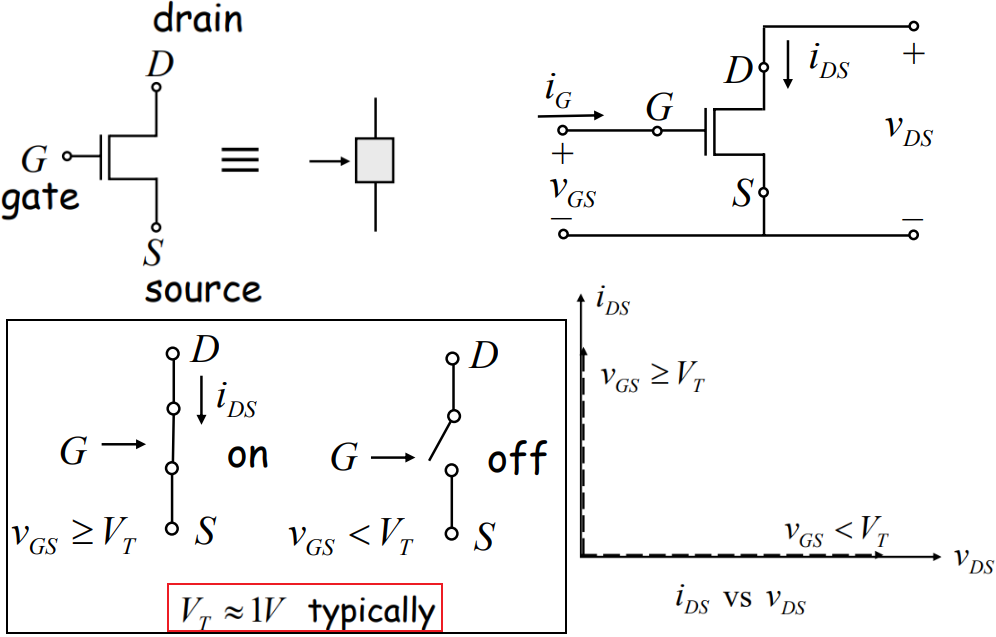MOSFET Inverter：The abstract inverter gate representation hides the internal details such as power supply connections, RL, GND, etc.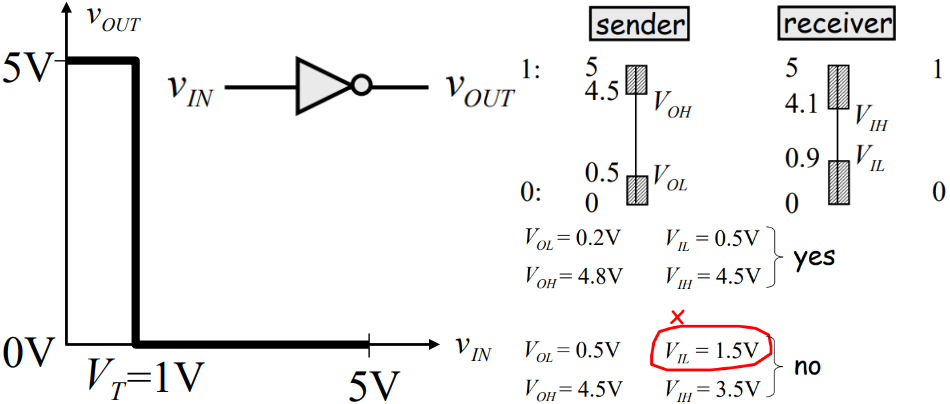• Vout是表示低电平的时候，输出要小于0.5 V(图中是0 V)，满足；
• Vout是表示高电平的时候，输出要大于4.5 V(图中是5 V)，满足；
• 有没有将所有低于VIL的视为低电平，是的(看横轴，低于0.9 V的对应的都是5 V低电平，反相之后即对应低电平)；
• 有没有将所有高于VIH的视为高电平，是的(看横轴，高于4.1 V的对应的都是0 V低电平，反相之后即对应高电平)；

Switch resistor modelSR model of the MOSFET，考虑了DS之间的电阻。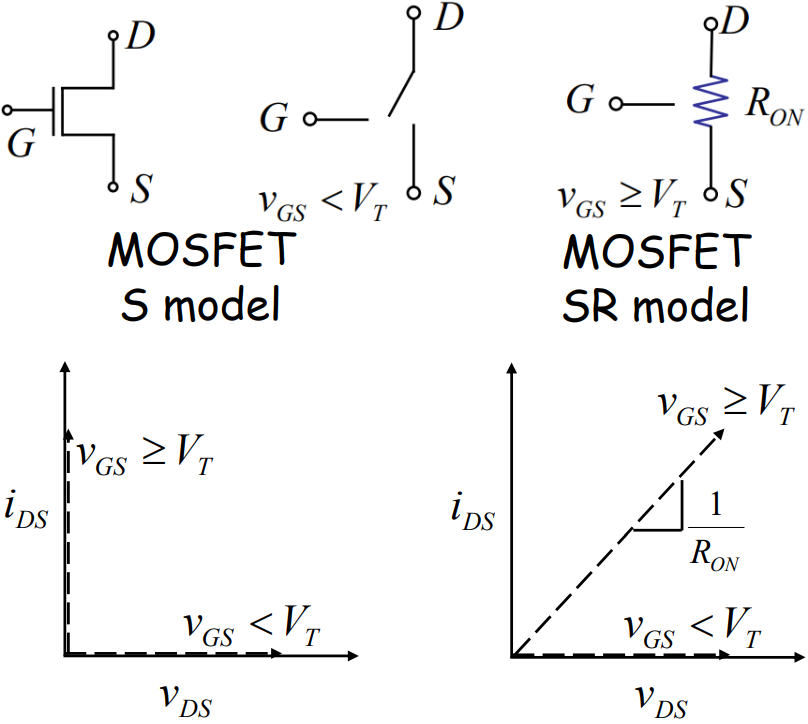SR model下之前研究的inverter的电路响应，如果要得到正确的输出，要满足什么条件？(下面左图的$$C=1$$)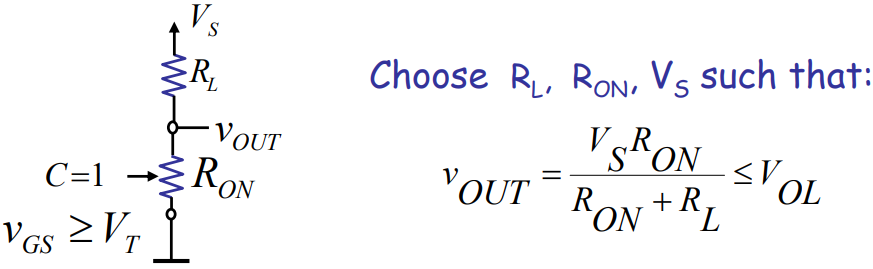(1) MOSFET Physics
(2) 三极管、场效应管、IGBT管的作用及区别

## Nonlinear analysis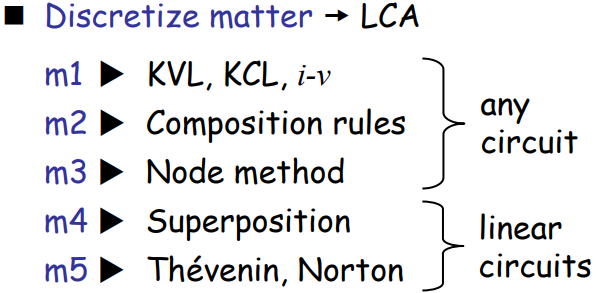How do we analyze nonlinear circuits, for example: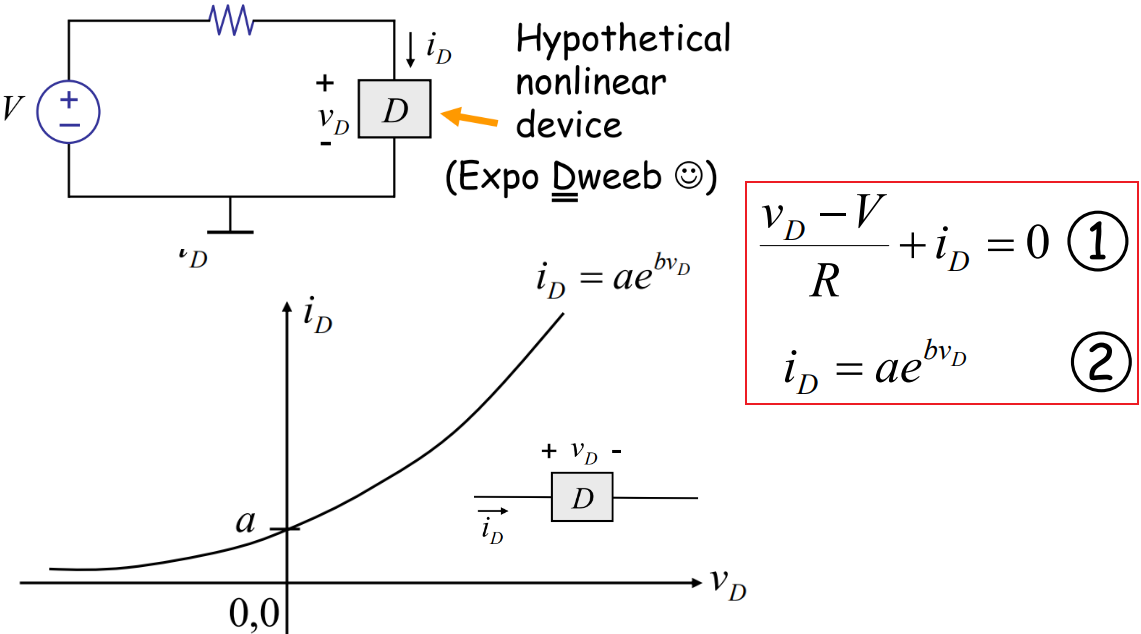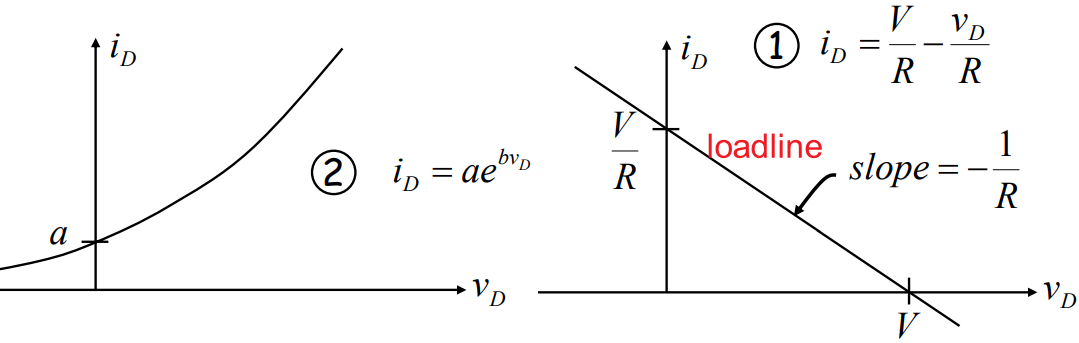piecewise linear method看课本4.4节。

Passive Devices——Components incapable of controlling current by means of another electrical signal are called passive devices. Resistors, capacitors, inductors, transformers, and even diodes are all considered passive devices.

Active Devices——An active device is any type of circuit component with the ability to electrically control electric charge flow (electricity controlling electricity). In order for a circuit to be properly called electronic, it must contain at least one active device. Active devices include, but are not limited to, vacuum tubes, transistors, silicon-controlled rectifiers (SCRs), and TRIACs.

Incremental Analysis(也叫small signal method)